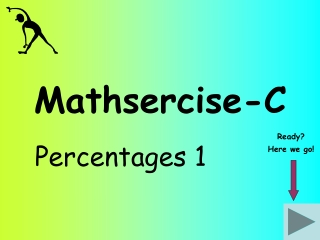DownloadDownload PresentationMathsercise-C

# Mathsercise-C

Télécharger la présentation## Mathsercise-C

- - - - - - - - - - - - - - - - - - - - - - - - - - - E N D - - - - - - - - - - - - - - - - - - - - - - - - - - -
##### Presentation Transcript

1. Mathsercise-C Percentages 1 Ready? Here we go!

2. Increase £350 by 10% Question 2 10% of 350 is 35 Remember 10% is one tenth 350 + 35 = £385 Answer 1 Percentages 1

3. Sale 20% off How much would a jacket priced at £90, cost in the sale? Question 3 20% of 90 is 18 Answer 90 - 18 = £72 2 Percentages 1

4. The cost of a CD player is £200 + vat. The rate of vat is 17.5%. What is the cost of the CD player? Question 4 VAT is easy to work out by hand if you do the following.. Answer 10% of 200 = 20 5% of 200 = 10 2.5% of 200 = 5 35 3 Percentages 1 200 + 35 = £235

5. A house cost £50,000 three years ago. I sold it for £90,000. What percentage profit have I made? Start 90,000 - 50,000 = £40,000 profit Now multiply by 100 to change it into percentage Answer 40,000 ÷ 50,000 = 0.8 0.8 x 100 = 80% 4 Percentages 1